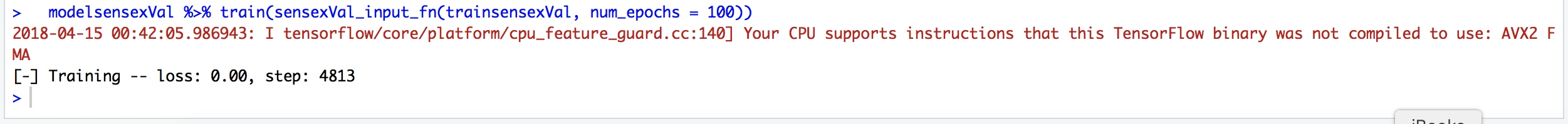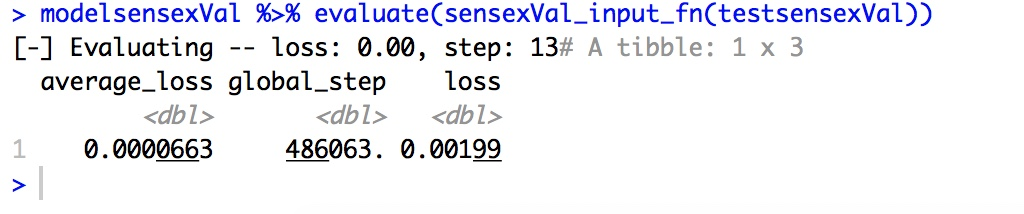Technical

# Programming a simple Predictor using TensorFlow and R

Programming a simple Predictor using TensorFlow and R: R Language provides interface for TensorFlow. Using this interface, it is possible to create very deep neural networks. However, to create quick and dirty and easy predictors, one can use the TensorFlow Estimators.

R Language provides interface for TensorFlow. Using this interface, it is possible to create very deep neural networks. However, to create quick and dirty and easy predictors, one can use the TensorFlow Estimators. I provide one simple example for the same.

The problem is to estimate the value of the Sensex Index based on the following parameters:

1. Historical prices of the Nifty
2. Historical prices of Gold
3. The date on which the above 2 prices were recorded
4. The day of the date
5. The month of the above date
6. The year of the above date
7. The day of the week of the date
8. The Julian day of the date

Before we start programming the predictor, let us look at the libraries that we require.

```if("lubridate" %in% rownames(installed.packages()) == FALSE) {install.packages("lubridate")}
library(lubridate)

if("tensorflow" %in% rownames(installed.packages()) == FALSE) {install.packages("tensorflow"); library(tensorflow); install_tensorflow()}
library(tensorflow)

if("tfestimators" %in% rownames(installed.packages()) == FALSE) {install.packages("tfestimators")}
library(tfestimators)
```

Next, let us load the data and have a look at the data.

```df <- read.csv(file="PriceIndex.csv", header=TRUE, sep=",")
df\$ObsDate <- as.Date(df\$ObsDate, "%Y-%m-%d")
df\$ObsMonth <- month(df\$ObsDate)
df\$YearNum <- year(df\$ObsDate)
df\$WeekDay <- as.POSIXlt(df\$ObsDate)\$wday
df\$ObsDay <- day(df\$ObsDate)
df\$ObsJulianDay <- as.numeric(format(df\$ObsDate, "%j"))
```

The first few records in the data.The last few records in the data.Now, we start building the model.

First, we need to set up the input function. The input function defines the independent variables and the dependent variables. In our case, the independent variable is the value of the Sensex. The dependent variables are values of Sensex, Gold, Date, Year, Month, Weekday and Julian Day.

```sensexVal_input_fn <- function(data, num_epochs = 1) {
input_fn(data,
features = c("ObsJulianDay", "ObsMonth", "ObsDay", "WeekDay", "YearNum", "Gold", "Nifty"),
response = "Sensex",
batch_size = 32,
num_epochs = num_epochs)
}
```

Next, we need to set up the feature columns. Feature Columns can be Categorical or Numeric. We use only Numeric columns in this example.

```sensexVal_cols <- feature_columns(
column_numeric("ObsJulianDay"),
column_numeric("ObsMonth"),
column_numeric("ObsDay"),
column_numeric("WeekDay"),
column_numeric("YearNum"),
column_numeric("Gold"),
column_numeric("Nifty")
)
```

Next, we need to set up the model. We set up a Linear Regressor. model_dir parameter specifies where the model will be stored on the disk. The stored model can be retrieved at a later point of time to make predictions using the same.

```modelsensexVal <- linear_regressor(feature_columns = sensexVal_cols, model_dir='./Value')
```

Next, we set up the data for training and testing. We use 90% of the available data for training and 10% of the available data for testing.

```dfModel <- subset(df, ObsDate <= as.Date(cutOfDate, "%Y-%m-%d"))
indicessensexVal <- sample(1:nrow(dfModel), size = 0.90 * nrow(dfModel))
trainsensexVal <- df[indicessensexVal, ]
testsensexVal  % train(sensexVal_input_fn(trainsensexVal, num_epochs = 100))
```Once the model has been trained, we can evaluate it.

```modelsensexVal %>% evaluate(sensexVal_input_fn(testsensexVal))
```Once the model has been created, it is persisted on the disk in the directory defined by the parameter model_dir.

We can write the following code to load the model if already available or generate the model if it is not created yet.

```# Assume the Sensex Model exists
generateSensexModel <- 0

# Check if the Sensex Model exists
if(file.exists("sensexValModelDir.rds")) {
modelsensexVal <- linear_regressor(feature_columns = sensexVal_cols,
model_dir = saved_SensexValmodel_dir)
if(is.null(modelsensexVal)) {
generateSensexModel <- 1
}
} else {
generateSensexModel <- 1
}

if(generateSensexModel == 1) {
modelsensexVal <- linear_regressor(feature_columns = sensexVal_cols, model_dir='./Value')

cutOfDate <- "2018-04-10"
dfModel <- subset(df, ObsDate <= as.Date(cutOfDate, "%Y-%m-%d"))
indicessensexVal <- sample(1:nrow(dfModel), size = 0.80 * nrow(dfModel))
trainsensexVal % evaluate(sensexVal_input_fn(testsensexVal))

# Save the model
saved_SensexValmodel_dir <- model_dir(modelsensexVal)
save(saved_SensexValmodel_dir, file="sensexValModelDir.rds")
}
```

Now that the model has been created, it can be used to predict the value of the independent variable.

```# Form the Prediction Parameters
ObsJulianDay <- c(as.numeric(format(TodayDate, "%j")))
ObsMonth <- c(month(TodayDate))
ObsDay <- c(day(TodayDate))
WeekDay <- c(as.POSIXlt(TodayDate)\$wday)
YearNum <- c(year(TodayDate))
Nifty <- c(YesterdayRecord\$Nifty)
Gold <- c(YesterdayRecord\$Gold)
obs <- data.frame(ObsJulianDay, ObsMonth, ObsDay, WeekDay, YearNum, Gold, Nifty)

# Predict Value
predictionValue % predict(sensexVal_input_fn(obs)))
predictionValue <- as.numeric(paste( unlist(predictionValue), collapse=''))
predictionValue
```

This site uses Akismet to reduce spam. Learn how your comment data is processed.